# EFFECT Function

Calculates the annual interest rate and the number of compounding periods per year

## What is the EFFECT Function?

The EFFECT function is categorized under Excel Financial functions. It will calculate the annual interest rate with the number of compounding periods per year. The effective annual interest rate is often used to compare financial loans with different compounding terms.

As a financial analyst, we often need to make decisions on which financial loan will be best for a company. The EFFECT function will be helpful in such scenario and will facilitate comparisons, ultimately helping in making a decision.

### Formula

=EFFECT(nominal_rate, npery)

The EFFECT function uses the following arguments:

1. Nominal_rate (required argument) – This is the nominal or stated interest rate.
2. Npery (required argument) – This is the number of compounding periods in one year.

### How to use the EFFECT Function in Excel?

To understand the uses of the EFFECT function, let’s consider a few examples:

#### Example

Suppose we are given the data below: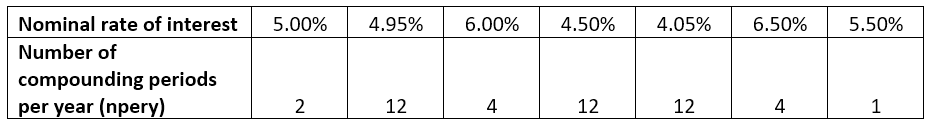The formula to use is:The result we get is the effective interest rate: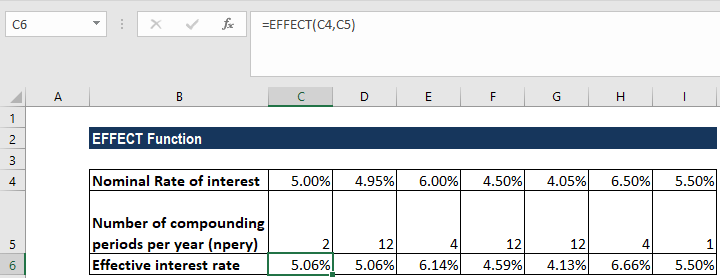Chances are that the result of the EFFECT function is displayed as a decimal or shows 0%. The issue is likely to be due to the formatting of the cell containing the function. It can be fixed by formatting the cell as a percentage with decimal places.

The different ways to format the cell are:

1. Use keyboard shortcut CTRL+1 (i.e., Select the CTRL key and while holding this down, select the “1” (one) key).

A format box would open up as shown below. Now, we can select Percentage from the category list on the left side of the dialog box.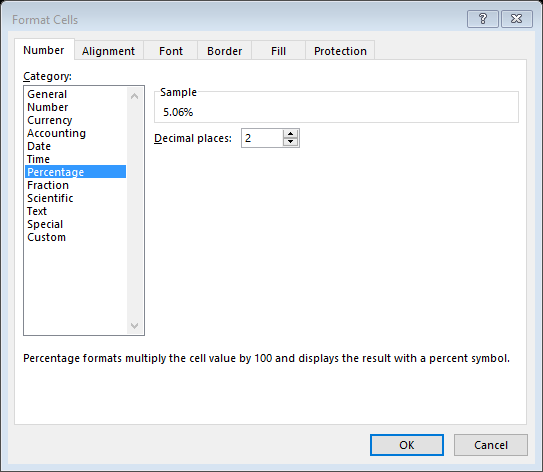It will open to more options on the righthand side of the control box, which allows us to select the number of decimal places that we want to be displayed. After selecting the desired number of decimal places, click OK.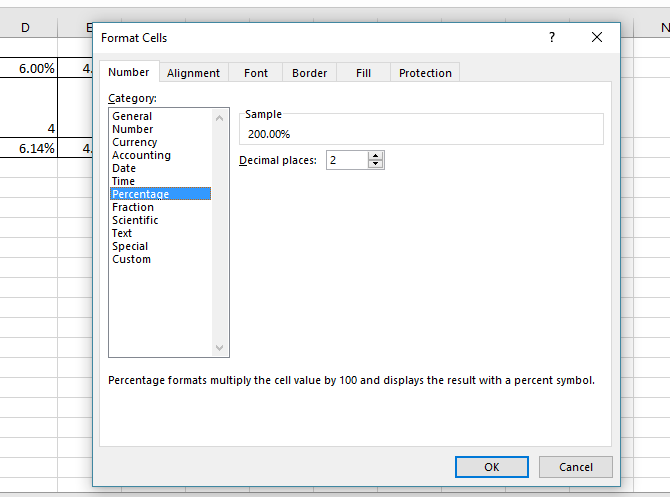1. The other method is to click the Home tab and select the range of cells on which the formatting will be done. Click on the Number tab and from the drop-down menu, select Percentage.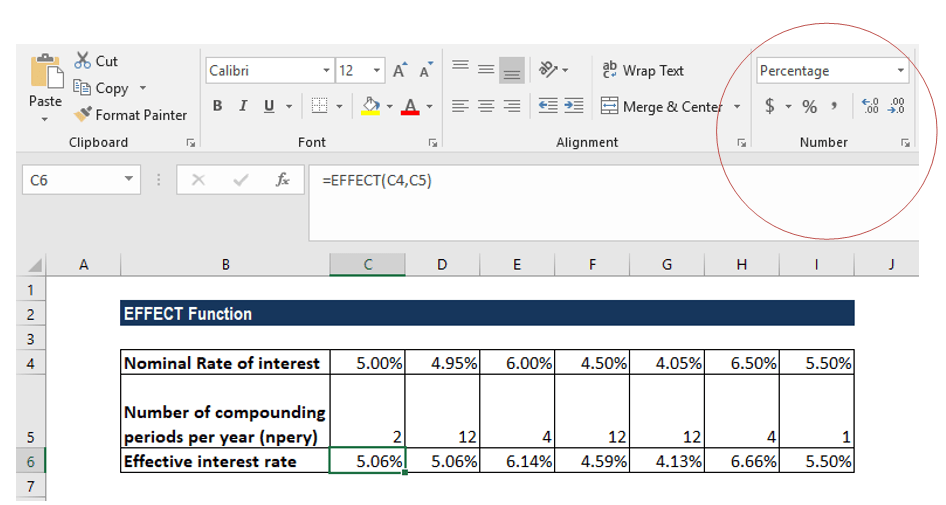EFFECT is calculated using the formula below: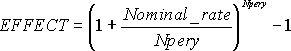Again, the nominal_rate is the nominal interest rate and npery is the number of compounding periods per year.

### Things to remember about the EFFECT Function

1. #VALUE! error – Occurs when:
• The nominal rate argument is not a numeric value.
• The npery argument is not a proper numeric value.
• Any of the arguments provided is non-numeric.
2. #NUM! error – Occurs when:
• The given nominal rate argument is less than or equal to 0.
• The given npery is less than or equal to 0.
3. If npery is in decimal format, it is truncated to integers.
4. The EFFECT function is related to the NOMINAL function through Effective rate = (1+(nominal_rate/npery))*npery – 1.

Thanks for reading CFI’s guide to the Excel EFFECT function. By taking the time to learn and master Excel functions, you’ll significantly speed up your financial analysis. To learn more, check out these additional CFI resources:

• Excel Functions for Finance